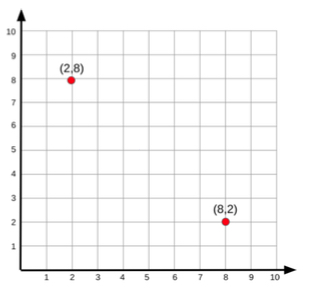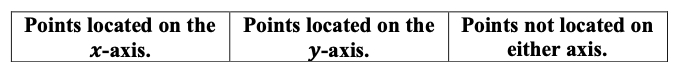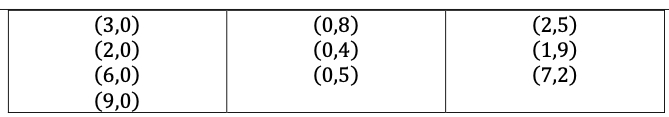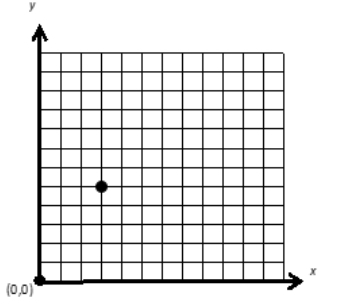# MA.5.GR.4.1Export Print
Identify the origin and axes in the coordinate system. Plot and label ordered pairs in the first quadrant of the coordinate plane.

### Clarifications

Clarification 1: Instruction includes the connection between two-column tables and coordinates on a coordinate plane.

Clarification 2: Instruction focuses on the connection of the number line to the x- and y-axis.

Clarification 3: Coordinate planes include axes scaled by whole numbers. Ordered pairs contain only whole numbers.

General Information
Subject Area: Mathematics (B.E.S.T.)
Strand: Geometric Reasoning
Status: State Board Approved

## Benchmark Instructional Guide

• Origin
• x-axis
• y-axis

### Vertical Alignment

Previous Benchmarks

Next Benchmarks

### Purpose and Instructional Strategies

The purpose of this benchmark is for students to extend their thinking from grade 4 (MA.4.NSO.1.3) about horizontal and vertical number lines to plot and label whole number ordered pairs on a coordinate plane. In addition, students will make a connection between a two- column table and the ordered pairs represented on the coordinate plane. In grade 6 (MA.6.GR.1.1), students plot rational number pairs in all four quadrants of the coordinate plane.
• During instruction, teachers should relate the coordinate plane as the intersection of two axes – a horizontal number line called the ??-axis and a vertical number line called the y- axis. The number lines that form the axes are perpendicular and meet at the origin, labeled by the ordered pair (0, 0) (MTR.5.1).
• When students learn to plot ordered pairs represented in a two-column table, they should understand that the ordered pair (x, y) represents how far to travel from the origin along the x- and y-axes.
• For example, students should understand that in the ordered pair (2, 4), the point travels along the x-axis 2 whole units to the right, and then vertically (parallel to the y-axis) 4 units up (MTR.5.1).

### Common Misconceptions or Errors

• Students can confuse the x- and y-values in an ordered pair and move vertically along the y-axis before moving horizontally along the x-axis.
• For example, they may mean to plot and label the ordered pair (2, 4), but plot and label (4, 2) instead. To assist students with this misconception, have students practice with creating directions for their student peers to follow to allow them to gain a better understanding of the direction and distance on the coordinate plane.
• Some students may not understand what an x- or y-coordinate value of 0 represents. During instruction, students should justify why ordered pairs with a 0 will plot on the x- axis or y-axis.

### Strategies to Support Tiered Instruction

• Instruction includes the teacher providing coordinate points to graph in quadrant 1 of the coordinate plane along with two small objects. The students explain how they move the object along the x-axis and then up the y-axis to the location provided. The teacher then provides the points reversed to graph and has students explain the difference in how they move the second object compared to the first.
• For example, the teacher provides students with a coordinate plane like the one shown below. The teacher provides a set of coordinate points such as (8,2). Students take turns moving an object, such as a two-colored counter, and explain the location of the point using the x- and y-axis in their explanation. The teacher then provides the points in reverse, (2,8). The next student will move a second object and explain the location of the point as well as the difference between the two locations.• Instruction includes the teacher providing a set of cards that have coordinate points on them, some with 0 as the location on the x-axis, some with 0 as the location on the y- axis, others with no 0 in the coordinates. Students sort the cards into three categories: points located on the x-axis, points located on the y-axis and neither. Students will justify their reasoning by explaining how the 0, or lack of a 0, in each set of points helped them.
• For example, the teacher provides cards with the following points on them: (2,5), (0,8), (3,0), (2,0), (1,9), (0,4), (6,0), (7,2), (9,0), (0,5) Students sort the points into three categories as shown below.• Instruction includes the teacher creating a giant coordinate plane on the floor with painters' tape or outside with sidewalk chalk. The teacher or a student will then create directions for their peers to follow. The teacher or student will provide a set of coordinate points, including those with 0 as the x- or y-coordinate. Another student will physically move to the location, describing as they move, which axis they are moving on and counting the spaces until they reach their final location.
• For example, the teacher or a student tells a student to move to the location of (4,6) on the coordinate plane. The student says, “I begin at the origin which is (0,0) and move 1, 2, 3, 4 spaces to the right on the x-axis. I then move 1, 2, 3, 4, 5, 6 spaces up on the y-axis to my final location of (4,6).”
• For example: The teacher provides a student with the location (5,0). The student will move along the x-axis 5 spaces and stop. The teacher provides another student with the location (0,5). That student moves up the y-axis 5 spaces and stop. The teacher will then have the students explain how their location ended up on the x- or y-axis as well as the relationship between those located on the y-axis and those located on the y-axis.

Part A. A point has coordinates (3, 5). If you were to graph this point on a coordinate plane, what does the 3 tell you to do?
Part B. Consider the same point with coordinates (3, 5). What does the 5 tell you to do?Part C. The point above has coordinates (3, 5). Which of these is the x-coordinate? Which of these is the y-coordinate?

### Instructional Items

Instructional Item 1

What ordered pair represents the origin of a coordinate plane?
• a. (0, 0)
• b. (1, 0)
• c. (0, 1)
• d. (1, 1)

Instructional Item 2

A point has coordinates (1, 6). If you were to plot this point on a coordinate plane, what does the 1 tell you to do?
• a. From the origin, move along the x-axis 1 unit up.
• b. From the origin, move along the y-axis 1 unit up.
• c. From the origin, move along the x-axis 1 unit right.
• d. From the origin, move along the y-axis 1 unit right.

*The strategies, tasks and items included in the B1G-M are examples and should not be considered comprehensive.

## Related Courses

This benchmark is part of these courses.
5012070: Grade Five Mathematics (Specifically in versions: 2014 - 2015, 2015 - 2022, 2022 and beyond (current))
7712060: Access Mathematics Grade 5 (Specifically in versions: 2014 - 2015, 2015 - 2018, 2018 - 2022, 2022 and beyond (current))
5012065: Grade 4 Accelerated Mathematics (Specifically in versions: 2019 - 2022, 2022 and beyond (current))
5012015: Foundational Skills in Mathematics 3-5 (Specifically in versions: 2019 - 2022, 2022 and beyond (current))

## Related Access Points

Alternate version of this benchmark for students with significant cognitive disabilities.
MA.5.GR.4.AP.1: Explore the first quadrant of the coordinate plane including the origin, axes and points located by using ordered pairs.

## Related Resources

Vetted resources educators can use to teach the concepts and skills in this benchmark.

## Educational Games

BattleGraph:

A game that is an off-shoot of the classic game Battleship, for practice with coordinate graphing, complete with reproducible templates and animated PowerPoint introduction.

Type: Educational Game

Maze Game:

In this activity, students enter coordinates to make a path to get to a target destination while avoiding mines. This activity allows students to explore Cartesian coordinates and the Cartesian coordinate plane. This activity includes supplemental materials, including background information about the topics covered, a description of how to use the application, and exploration questions for use with the java applet.

Type: Educational Game

## Formative Assessments

Understanding Coordinates:

Students are asked to explain the meaning of each coordinate and identify the x- and y-coordinates of a given ordered pair.

Type: Formative Assessment

On the Coordinate Plane:

Students are asked to graph points in the first quadrant of the coordinate plane.

Type: Formative Assessment

Properties of Coordinate Planes:

Students describe the different components of a coordinate plane and then determine the coordinates of a point located in quadrant one.

Type: Formative Assessment

Coordinates:

Students will use directions provided to locate a point on the coordinate plane and then identify its x- and y-coordinates.

Type: Formative Assessment

What Do the Coordinates Mean?:

Students are asked to explain the meaning of each coordinate and identify the x- and y-coordinates of a given ordered pair.

Type: Formative Assessment

## Lesson Plans

Plot the Constitution:

Students will work with a landscape architect to develop a plan for fountain placements and a walking path for a new city park. To complete the task, students must analyze and rank the U.S. Constitution‘s Bill of Rights according to the client’s directives. Students will apply geometry concepts to accurately plot and label coordinates on a park map. Throughout the process, students will work collaboratively to analyze and discuss their reasoning in this model eliciting activity.

Type: Lesson Plan

Voter Turnout and the 19th Amendment:

Students will graph and analyze voting data to explain how the 19th Amendment expanded civic participation in this lesson plan.

Type: Lesson Plan

Voter Turnout and the 15th Amendment:

In this lesson plan, students will graph and analyze voting data to explain how the 15th Amendment expanded civic participation.

Type: Lesson Plan

Hop on the Grid:

In this lesson students will learn how to plot ordered pairs on a grid. The students will physically hop on a (12x12) coordinate grid created by the teacher. The student will accurately plot ordered pairs given.

Type: Lesson Plan

Plotting for Treasure:

In this lesson, students will identify and graph points on the first quadrant of a coordinate plane and then use their knowledge to create and play a game to determine the position of treasure as indicated on a coordinate plane.

Type: Lesson Plan

Human Ordered Pairs:

This is a fun hands-on and interactive lesson on graphing ordered pairs on a coordinate grid.

Type: Lesson Plan

Graphing points on the coordinate plane.:

This lesson introduces the students to the coordinate system.

Type: Lesson Plan

Cartesian Classroom:

The classroom is turned into a human Cartesian coordinate plane, thereby introducing students to the characteristics of the Cartesian coordinate system.  The example given is for all 4 quadrants but the 5th grade standard may be addressed by designing the classroom as only the first quadrant.  In this case, questions regarding negative numbers would not be asked.

Type: Lesson Plan

"Design A Town" Coordinates:

Students will learn how to plot coordinates on the x and y-axis in the first quadrant. Students will start with plotting and creating simple shapes and progress through more complex shapes. The wrap up lesson will have the students plot and create their own town.

Type: Lesson Plan

Students will work cooperatively using varying modalities in order to demonstrate how to graph coordinates(ordered pairs) on a coordinate plane. Students will be engaged in singing, dancing, and using either stickers or self created illustrations to create a coordinate grid treasure map.

Type: Lesson Plan

## Original Student Tutorials

What Do You Mean the Coordinate Plane Doesn’t Fly?:

Learn about the basics of the coordinate plane by focusing on Quadrant I and see why the coordinate plane is useful in everyday life in this interactive tutorial.

Type: Original Student Tutorial

You Sank my Battleship! :

Learn how to accurately plot coordinates on a plane in interactive tutorial.

Type: Original Student Tutorial

Battle Ship Using Grid Paper:

The purpose of this task is to give students practice plotting points in the first quadrant of the coordinate plane and naming coordinates of points. It could be easily adapted to plotting points with negative coordinates. It also provides teachers with a good opportunity to assess how well their students understand how to plot ordered pairs and identify the coordinates of points.

## Tutorials

Coordinate Plane: Graphing Points in Quadrant I:

This Khan Academy tutorial video presents how to graph an ordered pair of positive numbers on the x- and y-axis of a coordinate plane.

Type: Tutorial

The Cartesian Coordinate System:

The Cartesian Coordinate system, formed from the Cartesian product of the real number line with itself, allows algebraic equations to be visualized as geometric shapes in two or three dimensions.  While this tutorial includes the basis of Coordinate system, it also includes ideas beyond fifth grade standards.  Most likely only advanced fifth graders would find the video engaging.

Type: Tutorial

## MFAS Formative Assessments

Coordinates:

Students will use directions provided to locate a point on the coordinate plane and then identify its x- and y-coordinates.

On the Coordinate Plane:

Students are asked to graph points in the first quadrant of the coordinate plane.

Properties of Coordinate Planes:

Students describe the different components of a coordinate plane and then determine the coordinates of a point located in quadrant one.

Understanding Coordinates:

Students are asked to explain the meaning of each coordinate and identify the x- and y-coordinates of a given ordered pair.

What Do the Coordinates Mean?:

Students are asked to explain the meaning of each coordinate and identify the x- and y-coordinates of a given ordered pair.

## Original Student Tutorials Mathematics - Grades K-5

What Do You Mean the Coordinate Plane Doesn’t Fly?:

Learn about the basics of the coordinate plane by focusing on Quadrant I and see why the coordinate plane is useful in everyday life in this interactive tutorial.

You Sank my Battleship! :

Learn how to accurately plot coordinates on a plane in interactive tutorial.

## Student Resources

Vetted resources students can use to learn the concepts and skills in this benchmark.

## Original Student Tutorials

What Do You Mean the Coordinate Plane Doesn’t Fly?:

Learn about the basics of the coordinate plane by focusing on Quadrant I and see why the coordinate plane is useful in everyday life in this interactive tutorial.

Type: Original Student Tutorial

You Sank my Battleship! :

Learn how to accurately plot coordinates on a plane in interactive tutorial.

Type: Original Student Tutorial

## Educational Game

Maze Game:

In this activity, students enter coordinates to make a path to get to a target destination while avoiding mines. This activity allows students to explore Cartesian coordinates and the Cartesian coordinate plane. This activity includes supplemental materials, including background information about the topics covered, a description of how to use the application, and exploration questions for use with the java applet.

Type: Educational Game

## Tutorials

Coordinate Plane: Graphing Points in Quadrant I:

This Khan Academy tutorial video presents how to graph an ordered pair of positive numbers on the x- and y-axis of a coordinate plane.

Type: Tutorial

The Cartesian Coordinate System:

The Cartesian Coordinate system, formed from the Cartesian product of the real number line with itself, allows algebraic equations to be visualized as geometric shapes in two or three dimensions.  While this tutorial includes the basis of Coordinate system, it also includes ideas beyond fifth grade standards.  Most likely only advanced fifth graders would find the video engaging.

Type: Tutorial

## Parent Resources

Vetted resources caregivers can use to help students learn the concepts and skills in this benchmark.

## Educational Game

BattleGraph:

A game that is an off-shoot of the classic game Battleship, for practice with coordinate graphing, complete with reproducible templates and animated PowerPoint introduction.

Type: Educational Game